#The Chain Rule

Suggested Prerequesites: The definition of the derivative

Suppose we want to find the derivative of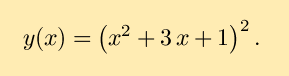We could hopefully multiply y(x) out and then take the derivative with little difficulty (in fact, this will be done below, as a check). But, what if, instead, the function were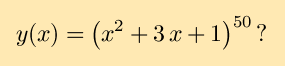Would you want to apply the same method to this problem? Probably not. Instead we need a method for dealing with composite functions, functions which are one function applied to another. For example, returning to the first y(x) above, if we let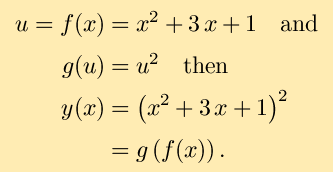The function y(x) is the composite of g with f.

Our goal is to find the derivative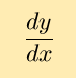based on our knowledge of the functions f and g. Now, we know that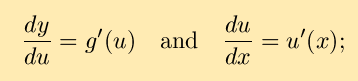Leibniz's differential notation suggests that perhaps derivatives can be treated as fractions, leading to the speculation that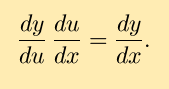This leads to the (possible) chain rule: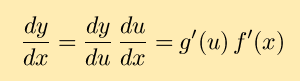Let's apply this to our example and see if it works. First, we'll multiply the product out and then take the derivative. Then we'll apply the chain rule and see if the results match: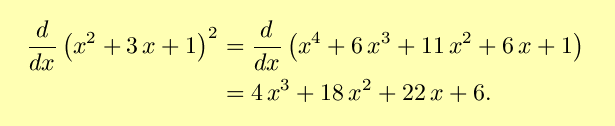Using the chain rule as explained above,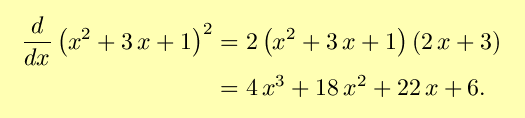So, our rule checks out, at least for this example. It turns out that this rule holds for all composite functions, and is invaluable for taking derivatives. For a more rigorous proof, see The Chain Rule - a More Formal Approach.

This rule is called the chain rule because we use it to take derivatives of composties of functions by chaining together their derivatives. The chain rule can be thought of as taking the derivative of the outer function (applied to the inner function) and multiplying it times the derivative of the inner function. The chain rule is arguably the most important rule of differentiation. It is commonly where most students tend to make mistakes, by forgetting to apply the chain rule when it needs to be applied, or by applying it improperly. Try to keep that in mind as you take derivatives.

### Some examples:

1. The function sin(2x) is the composite of the functions sin(u) and u=2x. Then,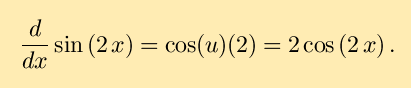2. An example that combines the chain rule and the quotient rule: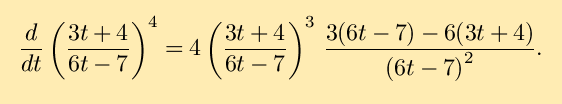(The fact that this may be simplified to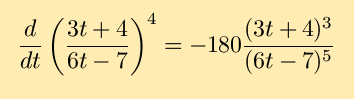is more or less a happy coincidence unrelated to the chain rule.)
3. The chain rule can be extended to composites of more than two functions. For example sin2(4x) is a composite of three functions; u2, u=sin(v) and v=4x. Just remeber, the derivative of the outer times the derivative of the inner times ... , giving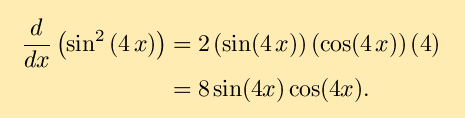As a check, you may want to note that the above may be expressed as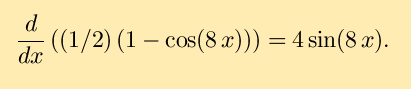### Exercises:

Differentiate the following with respect to the given independent variable: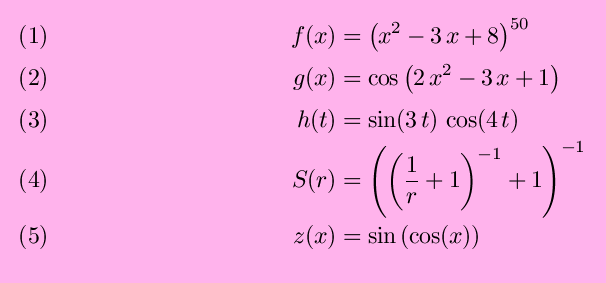Solutions to the exercises | Back to the Calculus page | Back to the World Web Math top page

watko@mit.edu